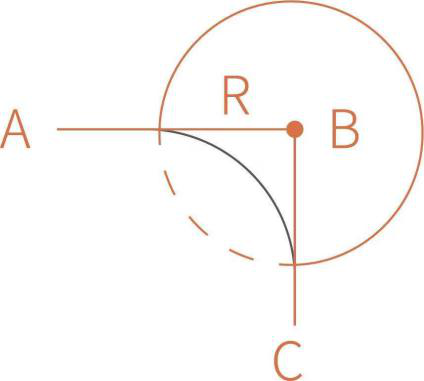# How to plan continuous movement？

Planning continuous motion must meet：
(1) xArm needs to use circular arc linear motion instruction when doing TCP motion, xArm’s joint instruction currently cannot plan continuous motion.
(2) Radian： R≥0, wait = False (do not wait). It is generally recommended that R> 0.

Figure 1 (∠ABC = 90 °)Figure 2) (∠ABC is any angle)

The above picture is an example. If you set R = 0, the speed of Lineb is continuous, and the turning speed at point B is 500mm / s. The turning effect is very sharp, and the effect is similar to the rigid body collision rebound. Above: ∠ABC = 90 °, from A to B to C, the speed is 500mm / s. It can be seen that at point B, the speed from A to B will be reduced to zero, and the speed from B to C will increase to 500mm / s, the theoretical acceleration for deceleration and acceleration is infinite. So it is not recommended that R = 0. Setting R> 0 will get a relatively smooth turning effect. The parameter （wait = fales） is set to accept the next instruction for planning. If wait = True, you need to wait.
(3) The robotic arm does not move immediately after receiving the instruction. It is necessary to set a waiting time and wait for multiple instructions to plan together. A single instruction cannot be continuous. The python interface is set_pause_time.

Blockly code example：

Python code example：
import os
import sys
import time

sys.path.append (os.path.join (os.path.dirname (file), ‘… /… /…’))

from xarm.wrapper import XArmAPI

arm = XArmAPI (‘192.168.1.221’)
arm.motion_enable (enable = True)
arm.set_mode (0)
arm.set_state (state = 0)

arm.reset (wait = True)
speed1 = 200

arm.set_pause_time (0.5)
while True:
arm.set_position (x = 400, y = -100, z = 250, roll = 180, pitch = 0, yaw = 0, radius = 50, speed = speed1, wait = False)
print (arm.get_position (), arm.get_position (is_radian = True))
arm.set_position (x = 400, y = 100, z = 250, roll = 180, pitch = 0, yaw = 0, radius = 50, speed = speed1, wait = False)
print (arm.get_position (), arm.get_position (is_radian = True))
arm.set_position (x = 300, y = 0, z = 250, roll = -180, pitch = 0, yaw = 0, radius = 50, speed = speed1, wait = False)
print (arm.get_position (), arm.get_position (is_radian = True))